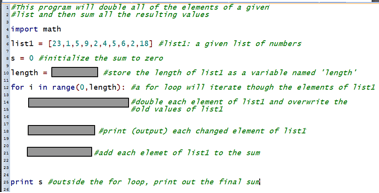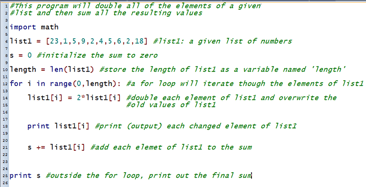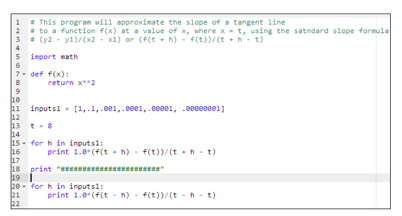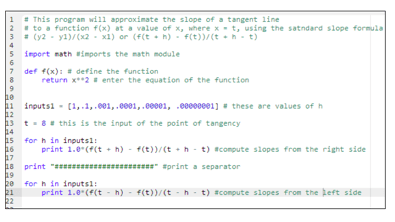# Hands-on ActivityPython Script Analysis

(0 Ratings)

### Quick Look

Grade Level: 12 (11-12)

Time Required: 45 minutes

Expendable Cost/Group: US \$0.00

This activity requires the use of non-expendable (reusable) computers or tablets; see the Materials List for details.

Group Size: 3

Activity Dependency:

Subject Areas: Computer Science, Problem Solving

### Summary

Working in small groups, students complete and run functioning Python codes. They begin by determining the missing commands in a sample piece of Python code that doubles all the elements of a given input and sums the resulting values. Then students modify more advanced Python code, which numerically computes the slope of a tangent line by finding the slopes of progressively closer secant lines; to this code they add explanatory comments to describe the function of each line of code. This requires students to understand the logic employed in the Python code. Finally, students make modifications to the code in order to find the slopes of tangents to a variety of functions.

### Engineering Connection

Engineers of all fields analyze systems, whether the electrical systems in automobiles, the chemical systems in petroleum extraction, river management systems, or the mechanical systems in airplanes. Software engineers must perform code analysis as part of the debugging process. Since computer code rarely performs correctly the first time it is written, analysis skills to determine the function of the code enable engineers to locate and solve any problems with the code. This activity provides a canvas for students to practice analysis of this type of system. They examine Python programming language code to determine what it does and make modifications based on their analyses, similar to what software engineers do daily to problem solve.

### Learning Objectives

After this activity, students should be able to:

• Use the Python programming language to investigate slopes of tangents.
• Analyze computer code to determine its function.
• Make changes to computer code based on their analyses.

### Educational Standards Each TeachEngineering lesson or activity is correlated to one or more K-12 science, technology, engineering or math (STEM) educational standards. All 100,000+ K-12 STEM standards covered in TeachEngineering are collected, maintained and packaged by the Achievement Standards Network (ASN), a project of D2L (www.achievementstandards.org). In the ASN, standards are hierarchically structured: first by source; e.g., by state; within source by type; e.g., science or mathematics; within type by subtype, then by grade, etc.

###### Common Core State Standards - Math
• Use function notation, evaluate functions for inputs in their domains, and interpret statements that use function notation in terms of a context. (Grades 9 - 12) More Details

Do you agree with this alignment?

• Understand the concept of a function and use function notation (Grades 9 - 12) More Details

Do you agree with this alignment?

###### International Technology and Engineering Educators Association - Technology
• Determine the best approach by evaluating the purpose of the design. (Grades 9 - 12) More Details

Do you agree with this alignment?

###### National Council of Teachers of Mathematics - Math
• Understand numbers, ways of representing numbers, relationships among numbers, and number systems (Grades Pre-K - 12) More Details

Do you agree with this alignment?

• Understand meanings of operations and how they relate to one another (Grades Pre-K - 12) More Details

Do you agree with this alignment?

###### National Science Education Standards - Science
• Communicate the problem, process, and solution. Students should present their results to students, teachers, and others in a variety of ways, such as orally, in writing, and in other forms — including models, diagrams, and demonstrations. (Grades 9 - 12) More Details

Do you agree with this alignment?

Suggest an alignment not listed above

### Subscribe

Get the inside scoop on all things TeachEngineering such as new site features, curriculum updates, video releases, and more by signing up for our newsletter!
PS: We do not share personal information or emails with anyone.

### Materials List

Each group needs:

### More Curriculum Like This

High School LessonPython Calculus

Students analyze a cartoon of a Rube Goldberg machine and a Python programming language script to practice engineering analysis. In both cases, they study the examples to determine how the different systems operate and the function of each component.

High School ActivityWhat is Python?

Students learn basic coding in Python, a high-level language that is known for its ease of use and various applications. They learn about the basic programming structures such as variables, objects, classes, and instances, and programming concepts such as if-else statements, loops, and functions.

### Pre-Req Knowledge

Teachers should have a working knowledge of the PythonTM computer language and know how to use one of the Python applications: Portable Python or Cloud9 IDE.

It is beneficial for students to have some computer programming knowledge, but it is not entirely necessary. Prior experience with Python is not required.

### Introduction/Motivation

Engineers are the ultimate problem solvers. The purpose of engineering is to find solutions to problems that exist. Pick any engineering discipline and you will find problem solvers. Structural engineers figure out how to span a gorge or build a building. Mechanical engineers are continually designing improvements to vehicles in order to address today's environmental challenges.

Part of problem solving is to analyze a problem or system and determine how it works so that potential changes can be made to improve or maintain it. In this activity, you will get practice with analysis of a system in the form of Python computer code.

### Procedure

Background

In this activity, students practice analyzing a problem in a computer code. The challenge is to analyze a Python code in two different ways. First, students look at the code with parts of it blocked out but with full comments; they must analyze the content to figure out the missing portions of the code. In the second task, they are given code with no comments. Again students must analyze the code, but in this case they add succinct descriptive and explanatory comments that would help someone without knowledge of Python understand what the code does. This process of analysis is similar to what software engineers do daily to problem solve.

Python was chosen as a platform for this activity because it is an introductory computer language that many high school students are exposed to in programming classes. From an engineering standpoint, calculus is often a tool that is employed. Since calculus is really a mathematical process that describes how something is changing, engineers of all different fields employ calculus to analyze changing systems.

Before the Activity

With the Students

1. Divide the class into groups of three students each. Give each group a handout, which contains two sets of codes. Direct students to focus on the code labeled "Step 1 Python code," also shown in Figure 1. Note that parts of certain lines of this code are blocked out.Figure 1. Step 1 Python code with some lines of code blocked out.copyrightCopyright © 2013 Scott Burns, University of Nebraska-Omaha
2. Give student groups some time to brainstorm and tap their prior experience to analyze the observable parts of the code and the comments to determine what the missing code is most likely to be. If students are unfamiliar with the Python commands, provide each group with a sheet that explains common Python commands and functions. Depending on students' level of programming experience, provide the necessary guidance to help them fill in the missing pieces of code, and explain the purpose and process of each line.
3. With the class, review the answer to the missing lines of code, as provided in the Python Calculus Activity Handout Answer Key, and in Figure 2. One way to do this is to project the blocked-out code on the classroom board and assign student groups to fill in the areas of missing code. Discuss the answers and clarify any confusion.Figure 2. The answer (missing code) to the Step 1 Python code problem.copyrightCopyright © 2013 Scott Burns, University of Nebraska-Omaha
4. Next, direct students to focus on the Step 2 Python code on their handouts, also shown in Figure 3. This is a completed Python code that computes the slope of a tangent line for a given function at a specified input. This code is lacking descriptive comments that explain the purpose of each line of code.Figure 3. Step 2 Python code without comments.copyrightCopyright © 2013 Scott Burns, University of Nebraska-Omaha
5. Inform student groups that the engineering challenge is to analyze the code and add appropriate comments that give very brief but accurate descriptions of the code purpose.Figure 4. The answer (missing comments) to the Step 2 Python code problem.copyrightCopyright © 2013 Scott Burns, University of Nebraska-Omaha
6. Once students have had time to develop detailed comments for each line of code, review the answer with the class. Again, it works well to project the code on the classroom board, to which student groups can write in in their comments on the board. Refer to the answer key or Figure 4 for the solution to this problem.
7. Conclude by administering the post-activity assessment, as described in the Assessment section.

### Vocabulary/Definitions

Python programming language: A general purpose, high-level computer language.

### Assessment

Pre-Activity Assessment

Questions: Ask students the following questions to gauge their prior knowledge.

• What is another way to describe the slope of a tangent line to a curve? (Answer: The slope of a tangent line to a curve is the derivative of the curve function at the point in which the tangent line intersects the curve. The slope also defines the rate of change of the curve at the point in which the tangent line intersects the curve.)
• Why is it important for computer programmers to include comments? (Answer: Comments are important so that the computer programmer can keep track of the function of each line or step of the code, and comments are also extremely useful if someone besides the original programmer wants to use or modify the code.)

Activity Embedded Assessment

Observations: As students are engaged in the activity, consider these or similar questions in order to assess their progress and understanding:

• Are students able to translate from human comments to Python code and from the code to comments?
• Are students able to alter working code in order to compute derivatives for new functions or new input values?

Post-Activity Assessment

Final Test: Administer the Python Calculus Activity Assessment, in which students must apply their understanding of the code that was analyzed in Steps 1 and 2 of the activity to determine derivatives to five functions in the form f(x) at specified values of x. The first four problems are straightforward and simply require that students replace the function and the value of x in the code. Direct student groups to divide up these problems, so that each member completes at least one and shares his or her results with the rest of the group. The fifth problem is more challenging because the function has a non-differentiable cusp at the provide x value, so direct groups to work together to determine why no derivative exists at the x value provided. Refer to the Python Calculus Activity Assessment Answer Key for more details.

© 2013 by Regents of the University of Colorado; original © 2013 Board of Regents, University of Nebraska

### Contributors

Scott Burns, Brian Sandall

### Supporting Program

IMPART RET Program, College of Information Science & Technology, University of Nebraska-Omaha

### Acknowledgements

The contents of this digital library curriculum were developed as a part of the RET in Engineering and Computer Science Site on Infusing Mobile Platform Applied Research into Teaching (IMPART) Program at the University of Nebraska-Omaha under National Science Foundation RET grant number CNS 1201136. However, these contents do not necessarily represent the policies of the National Science Foundation, and you should not assume endorsement by the federal government.